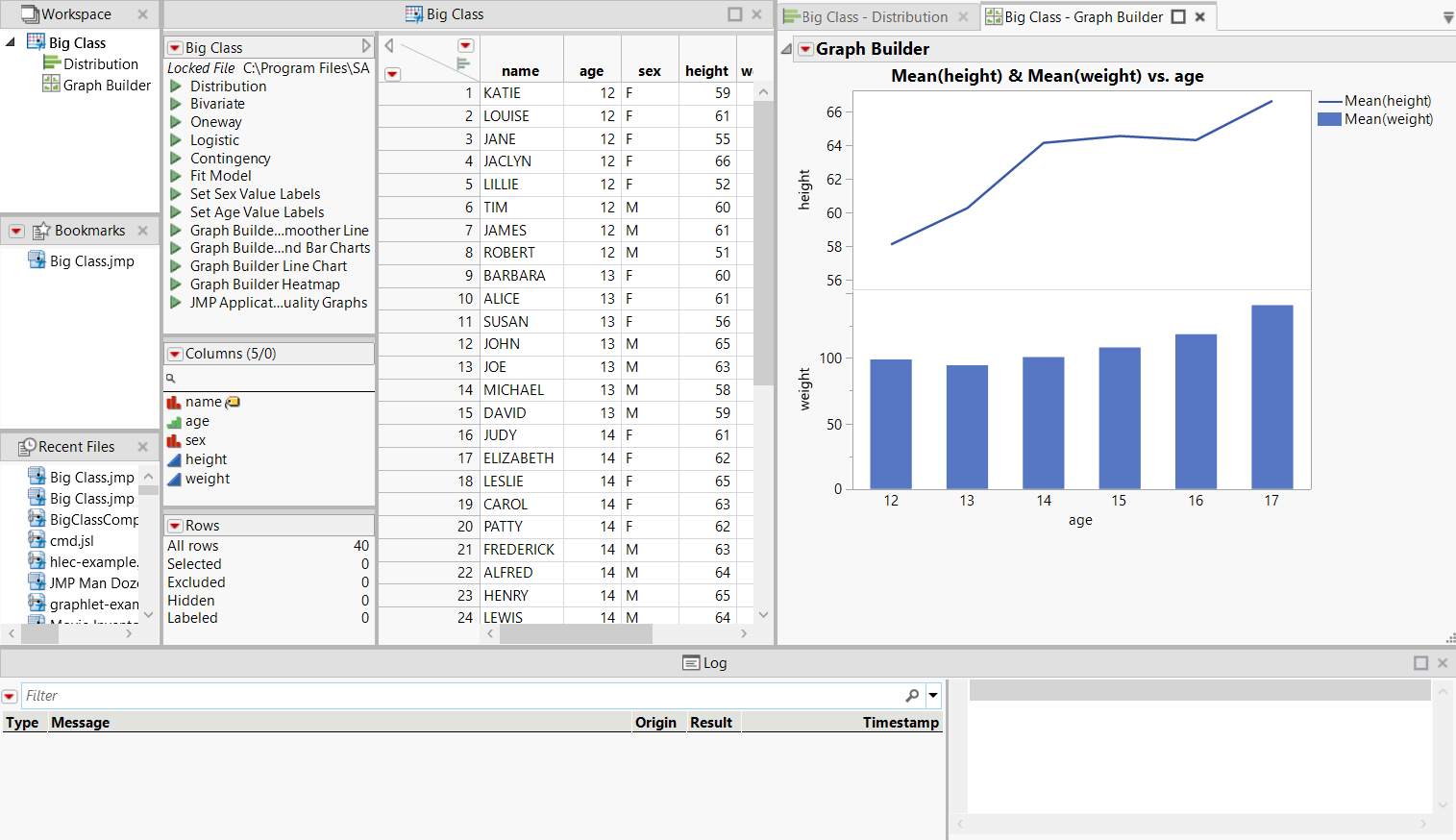Scripting Guide > Creating Projects > Create a Project with a Bookmarks Pane and Log
Publication date: 04/12/2021

# Create a Project with a Bookmarks Pane and Log

A more complicated project might include a Bookmarks pane (to show file, folders, and groups that use frequently), and a project log. The data table and output are in separate tabs.

Begin by creating a new project and creating a bookmark.

`project = New Project();`
`project << Add Bookmarks({`
`              File( "\$SAMPLE_DATA/Big Class.jmp" )`
`});`

Run Script opens the data table and creates output inside the project.

`project << Run Script(`
`	Open(`
`		"\$SAMPLE_DATA/Big Class.jmp",`
`		Set Window ID( "data" ) // unique window ID`
`	);`
`	Distribution(`
`		Set Window ID( "distrib" ),`
`		Continuous Distribution( Column( :weight ) ),`
`		Nominal Distribution( Column( :age ) )`
`	);`
`	Graph Builder(`
`		Set Window ID( "graphbuilder" ),`
`		Size( 443, 409 ),`
`		Show Control Panel( 0 ),`
`		Fit to Window( "Maintain Aspect Ratio" ),`
`		Variables( X( :age ), Y( :height ), Y( :weight ) ),`
`		Elements( Position( 1, 1 ), Line( X, Y, Legend( 1 ) ) ),`
`		Elements( Position( 1, 2 ), Bar( X, Y, Legend( 2 ) ) )`
`	);`
`);`

Set Layout specifies the layout of the tabs that show the data table and output.

`project << Set Layout(`
`		V Splitter Box(`
`			Size( 1215, 700 ),`
`			<<Set Sizes( {0.77, 0.23} ),`
`			<<Dockable(),`
`			V Splitter Box(`
`				Size( 1215, 540 ),`
`				<<Set Sizes( {1} ),`
`				<<Dockable(),`
`				H Splitter Box(`
`					Size( 1215, 540 ),`
`					<<Set Sizes( {0.11, 0.42, 0.47} ),`
`					<<Dockable(),`
`					V Splitter Box(`
`						Size( 128, 540 ),`
`						<<Set Sizes( {0.33, 0.33, 0.33} ),`
`						<<Dockable(),`
`						Tab Page Box(`
`							Title( "Window List" ), // show the Window List`
`							Window ID( "Window List" )`
`						),`
`						Tab Page Box(`
`							Title( "Bookmarks" ), // show the Bookmarks pane`
`							Window ID( "Bookmarks" )`
`						),`
`						Tab Page Box(`
`							Title( "Recent Files" ), // show the Recent Files pane`
`							Window ID( "Recent Files" )`
`						)`
`					),`
`					Tab Page Box( // show the data table`
`						Title( "Big Class" ),`
`						Window ID(`
`							"data"`
`						)`
`					),`
`					Tab Box(`
`						<<Dockable(),`
`						Tab Page Box( // show the Distribution graph and reports`
`							Title( "Big Class - Distribution" ),`
`							Window ID(`
`								"distrib"`
`							)`
`						),`
`						Tab Page Box( // show the Graph Builder graph`
`							Title( "Big Class - Graph Builder" ),`
`							Window ID(`
`								"graphbuilder"`
`							)`
`						)`
`					)`
`				)`
`			),`
`			Tab Page Box( Title( "Log" ), Window ID( "Log" ) )`
`		)`
`);`

Save saves the project.

// if the project has already been saved, the Save message

// with no argument saves the file to the existing location

`project << Save( "\$DOCUMENTS/Big Class.jmpprj" );`

Note: If you're saving a project with unsaved documents in JSL, the project doesn't close and you get a log message that the project has unsaved documents. All files in a project must be saved or closed before the project can be saved. However, if you interactively save a project with unsaved documents, you are prompted to save the documents.

Figure 15.2 Example of a Complex ProjectOpen the project from another script:

`project = Open( "Big Class.jmpprj" );`

In this example, the script that you are running to open the project is in the same folder as the project.

Want more information? Have questions? Get answers in the JMP User Community (community.jmp.com).